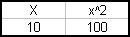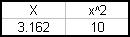## Trick 2 - Entering Numbers in Formulas

Here is a snippet from a sheet. X contains a number, and XX is X squared.i.e. The formula in cell X is "=X*X"Now type 10 in cell XX overwriting the formula, and you get this.

X now contains the square root of 10, and XX still contains the formula "=X*X"

What's going on?

Well, there is some code behind what is happening.

`Sub Start()      Application.OnEntry = "Update"End SubSub Update()On Error GoTo errorhandler    With Application.Caller        If Application.Caller.Address = Range("XX").Address Then            Range("X") = Sqr(Application.Caller)            Range("XX").Formula = "=X*X"        End If    End With    Exit Suberrorhandler:    Exit SubEnd Sub`

First the start macro needs to be run. Typically this would sit in the autoexec macro.

Then when something is entered on the sheet, the Update macro is called. This checks to see if you are overwritting the formula in XX. If it is, it calculates the square root, and repastes the formula back in cell XX.

End result, code that works both ways.

If there is a function such as eval in vba, the square root call could also be parameterised.